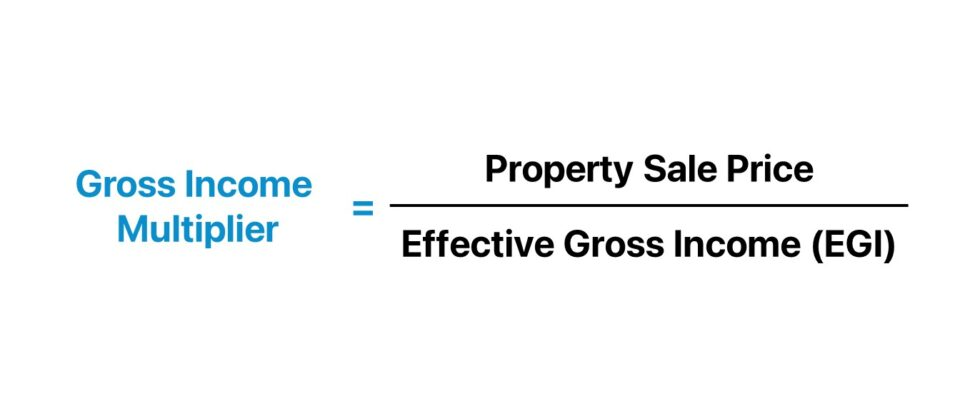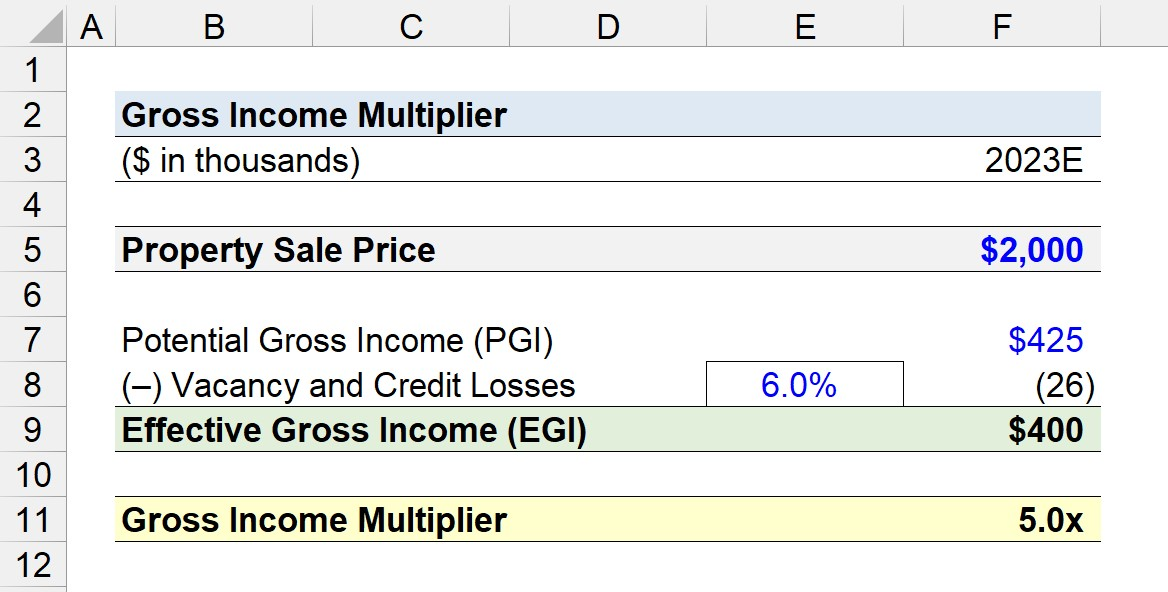Welcome to Wall Street Prep! Use code at checkout for 15% off.# Gross Income Multiplier (GIM)

Guide to Understanding Gross Income Multiplier (GIM)## How to Calculate Gross Income Multiplier?

The gross income multiplier (GIM) is a real estate valuation metric used to estimate the value of property investments.

Formulaically, the gross income multiplier is the ratio between the sale price of a property and its gross annual income.

In practice, the primary use-case of the gross income multiplier (GIM) is to arrive at an approximate market value for rental properties, in which the gross income component stems predominately from rent payments.

For rental properties, the investor recoups the initial project cost and earns a return from the income stream of rent payments collected from tenants.

The multiplier is used as a method to compare similar properties side-by-side, i.e. closer to an “apples-to-apples” comparison”.

The gross income multiplier calculation is a three-step process:

1. Identify Property Sale Price → The first step is to identify the sale price of the property, which is usually readily available online (i.e. the listing price). The stated sale price, at least in theory, should reflect the property’s fair value with room for some minimal deviation, but there can be exceptions, of course.
2. Estimate Gross Annual Income → The gross annual income can either be the potential gross income (PGI) or effective gross income (EGI) metric. The potential gross income (PGI) is computed under the assumption that all rentable units are occupied, i.e. at a 100% occupancy rate (or 0% vacancy rate). However, the effective gross income (EGI) – unlike PGI, which deducts credit and collection losses – is the income metric that is used more often.
3. Calculate Gross Income Multiplier (GIM) → In the last step, the gross income multiplier is determined by dividing the sale price by the effective gross income (EGI).

Note: The gross income metric must be annualized and presented on a stabilized basis.## Gross Income Multiplier Formula

The formula to calculate the gross income multiplier (GIM) metric divides the sale price of a property by its effective gross income (EGI).

Gross Income Multiplier (GIM) = Property Sale Price ÷ Effective Gross Income (EGI)

Where:

• Property Sale Price → The stated selling price of the property that is currently on the market for sale.
• Effective Gross Income (EGI) → The total income generated by the property across one year, prior to subtracting operating expenses.

The effective gross income (EGI) formula is the potential gross income (PGI), net of the credit and collection losses expected to be incurred by the property.

While the potential gross income (PGI) can be used as the income metric, the effective gross income (EGI) is perceived to be a more accurate measure of a property’s income potential.

Gross Income Multiplier (GIM) = Property Sale Price ÷ Potential Gross Income (PGI)

Note: The gross income multiplier (GIM) in which the effective gross income (EGI) metric is used, rather than the potential gross income (PGI), is often referred to as the “Effective Gross Income Multiplier (EGIM)”.

## Gross Rent Multiplier (GRM) vs. Gross Income Multiplier (GIM)

The terms “Gross Rent Multiplier” (GRM) and “Gross Income Multiplier” (GIM) are often used interchangeably, yet there is a minor distinction.

• Gross Rent Multiplier (GRM) → The gross rent multiplier (GRM) is the ratio between the market value of a property and its gross rental income. The GRM is most frequently used for residential properties, as the metric does not account for other forms of ancillary income, such as fees collected for amenities.
• Gross Income Multiplier (GIM) → In contrast, the gross income multiplier (GIM) is more inclusive since all forms of income generated by the property are factored into the metric rather than just rental income. Hence, the gross income multiplier is more frequently used in commercial properties with multiple streams of income.

Note: Many practitioners refer to the two metrics interchangeably, so it is necessary to confirm the formula.

## Gross Income Multiplier Calculator

We’ll now move on to a modeling exercise, which you can access by filling out the form below.#### Excel TemplateSubmitting...

## Gross Income Multiplier Calculation Example (GIM)

Suppose a commercial real estate (CRE) investor is considering the purchase of a commercial property currently listed for sale at \$2 million.

Based on historical performance and market analysis, the commercial property is expected to generate \$425k in effective gross income (EGI) in 2023 – which represents the first year in which the property is stabilized.

The \$425k in effective gross income (EGI) was derived from the pro forma assumption that the vacancy and credit losses would be around 6.0% of the property’s potential gross income (PGI).

• Property Sale Price = \$2 million
• Potential Gross Income (PGI) = \$425k
• Effective Gross Income (EGI) = \$425k – (6.0% × \$425k) = \$400k

The effective gross income (EGI) of the commercial property is \$400k, which we’ll insert into our formula to arrive at a gross income multiplier of 5.0x.

• Gross Income Multiplier (GIM) = \$2 million ÷ \$400k = 5.0x

Given the gross income multiplier of 5.0x, the commercial property must continue to generate the current run rate of effective gross income (\$400k) for approximately 5 years to recoup the original project cost, ignoring the operating expenses incurred while managing the property.20+ Hours of Online Video Training

### Master Real Estate Financial Modeling

This program breaks down everything you need to build and interpret real estate finance models. Used at the world's leading real estate private equity firms and academic institutions.

Inline Feedbacks